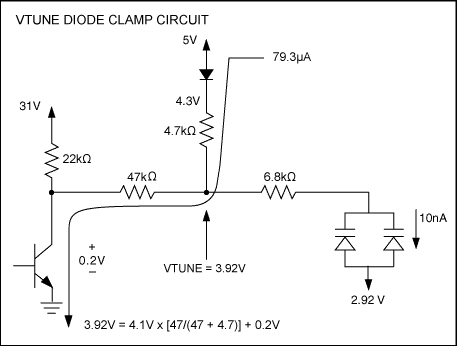# Preventing Parasitic Mode Oscillation in MAX2104 Circuits

### 要約

The best way to eliminate parasitic mode oscillation during MAX2104 start up is to program to an initial frequency that results in a large value for Vtune, bringing the tune voltage above the clamp voltage. This application note describes how.

### Introduction

The following article explains why toggling the frequency doubler will remove the parasitic oscillation-mode observed with the MAX2104.

The parasitic oscillation is encountered when the varactor Vtune voltage drops below 3.9V. The loaded tank Q is very low at this point, and the oscillator then finds a very high-Q parasitic resonant path. This path is typically a series resonance path consisting of a very low loss series inductance resonating with the internal capacitance of the oscillator cell. It is this very high-Q series resonance path which dominates the parasitic mode oscillation at low tuning voltages. Series resistors (20Ω) are often used to de-Q the parasitic mode series resonance.

During normal operation, a diode clamp circuit is utilized to maintain Vtune above 3.92V (See Figure 1). This clamp circuit in the existing application circuit is implemented to prevent the tank from entering parasitic mode during normal operation.Figure 1. Typical diode clamp circuit.

However, the parasitic mode problem is most problematic during the initial power up sequence. Experimentally, it has been shown several times that toggling the LO doubler during start up solves the parasitic mode oscillation. The following analysis will explain why toggling the LO doubler solves the parasitic oscillation mode.

Figure 1 shows during worst case steady-state operation where the charge pump transistor becomes saturated. Vtune is then clamped to 3.92V to keep the oscillator out of its parasitic oscillation mode.

### Analysis

For example, lets assume the set top box is initially programmed to lock at 1458MHz with the LO doubler on. In an ideal situation the PLL would operate normally as shown in Figure 2.Figure 2. Normal PLL operation.

In normal operation, the comparison frequency for the crystal and the local oscillator is 625kHz, Vtune is around 10.3V, and the local oscillator is oscillating at 729MHz. This frequency is doubled in the doubler circuitry to 1458MHz. An equivalent divide ratio of N = 2332.8 is programmed. The charge pump is in equilibrium, and the charge pump current is pumped up and down as needed.Figure 3. Parasitic mode oscillation.

In parasitic mode oscillation, the local oscillator is oscillating at approximately 1410MHz. With the LO doubler on, this frequency is doubled to 2820MHz. This produces an LO comparison frequency of 1208kHz. Since this is larger than the crystal comparison frequency of 625kHz the charge pump will pump down as much as possible to lower the tune voltage. This positive feedback keeps the tune voltage as low as it will go. With the tune voltage at a minimum, it is impossible to exit the parasitic resonant mode since the loaded tank Q is at a minimum for the desired fundamental mode oscillation.Figure 4. Toggle LO doubler.

Figure 4 shows PLL operation when the LO doubler is toggled. Now that the LO is no longer doubled, the LO comparison frequency drops to 604kHz. Since this is below the crystal reference comparison frequency of 625kHz, the charge pump pumps up and increases the tune voltage. The tune voltage will climb from 3.8V to 31V. When Vtune rises from 3.8V to 31V the loaded Q of the tank improves enough to dominate and oscillate in fundamental mode.Figure 5. Parasitic mode cure.

Figure 5 shows the resultant exit from parasitic mode. Vtune is forced to 30V due to positive feedback. The charge pump is in a continuous pump up mode. The maximum oscillation frequency for the tank circuit is reached, around 1160MHz. At this point in time if the doubler is activated the PLL will return to normal operation shown in Figure 1.

### Conclusion

From the analysis above several conclusions are drawn:

1. The best way to eliminate parasitic mode oscillation during start up is to program to an initial frequency that results in a large value for Vtune. This will immediately get the tune voltage above the clamp voltage. When this is achieved the "Vtune diode clamp voltage" circuit will keep the tune voltage above the point where the tank is susceptible to parasitic mode operation.
2. Toggling the frequency doubler will remove the parasitic mode oscillation.